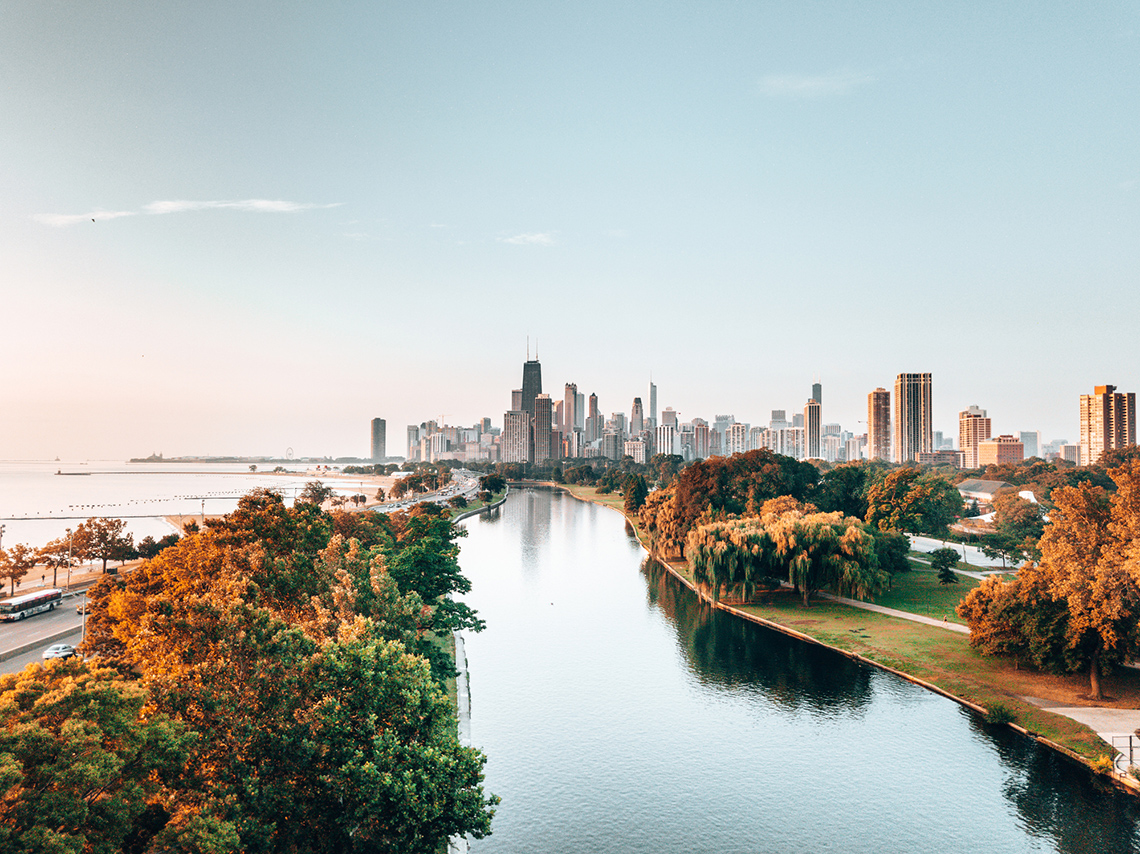# Minds On

## What’s the area?

Examine the following rectangles. Which one has a greater area? How do you know?

Record your ideas in a notebook or a method of your choice.Press ‘Hint’ for a tip about area.

Area is the entire surface of the shape.

# Action

## Arrays

In the Minds On section, we explored two rectangles that were arranged as arrays.

An array can consist of objects or square units.

The following image displays some examples of arrays.When we measure the area of a rectangle, the surface of the shape must be covered by units of area without any gaps or overlap. These units of area are called square units.

Instead of counting each square unit in an array to find the area, we can identify the base length, and multiply this by the height to find the total area in square units.

These square units as metric units of area are square centimetres (cm²) and square metres (m²).

### Array example

Let’s determine which of the following rectangles has a greater area by using the array. Each square is 1 square centimetre (cm²).We can use the numbered grid to help us identify the base length and height.

Let’s begin with the taller rectangle.

1. The base length of the taller rectangle is 7 centimetres. The height of this rectangle is 5 centimetres.
• To find the total square units, we multiply our base length with the height.
• 7 cm × 5 cm = 35 cm²
• The total area of the taller rectangle is 35 square centimetres.

Now, we’ll find the area of the longer rectangle.

1. The base length of the longer rectangle is 12 square units. The height is 3 square units.
• 12 cm × 3 cm = 36 cm²
• Therefore, the longer rectangle has a greater area than the other rectangle.

## Task 1: Using arrays to find area

Solve for the area of the following rectangles. You can record your thinking using a method of your choice.When you’re ready, press ‘Answer’ to reveal the area of the rectangle.

The base length is 2 centimetres. The height is 8 centimetres.

2 × 8 = 16

The area of the rectangle is 16 square centimetres (cm²).When you’re ready, press ‘Answer’ to reveal the area of the rectangle.

The base length is 8 centimetres. The height is 8 centimetres.

8 × 8 = 64

The area of the rectangle is 64 square centimetres (cm²).When you’re ready, press ‘Answer’ to reveal the area of the rectangle.

The base length is 8 centimetres. The height is 4 centimetres.

8 × 4 = 32

The area of the rectangle is 32 square centimetres (cm²).

# Consolidation

## Which area is greater?

Student A and Student B each have a piece of graph paper. They need to determine which piece has the larger area to use for a project

Use your understanding of arrays to find the area of both rectangles.

Record your ideas in a notebook or a method of your choice.

The following is student A’s graph paper:The following is student B’s graph paper:• Can we use arrays or part of an array to help us find the area of different objects?
• How do arrays help us to understand the area of a rectangle?

## Reflection

As you read through these descriptions, which sentence best describes how you are feeling about your understanding of this learning activity? Press the button that is beside this sentence.### I feel...

Now, record your ideas using a voice recorder, speech-to-text, or writing tool.

Press ‘Discover More’ to extend your skills.

### City arraysLet’s explore the following example of a cityscape. Many cityscapes include rectangular structures of different heights and sizes.Use the following printable grid paper to create your own cityscape. You can also complete your cityscape in your notebook or using another method of your choice.

You can use a variety of different shapes, but you must include 3 arrays:

• base length of 3 square units, and a height of 8 square units
• base length of 5 square units, and a height of 4 square units
• base length of 2 square units, and a height of 6 square units

Calculate the area of each array on the printable grid template or use another method of your choice to record your work.

Press ‘Array City Example’ to reveal an example of a city that uses arrays.Complete the City Arrays activity in your notebook or using the following printable document. You can also use another method of your choice.